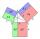# Medians of isosceles triangle

The isosceles triangle has a base ABC |AB| = 16 cm and 10 cm long arm. What are the length of medians?

Result

t1 =  6 cm
t2 =  12.369 cm
t3 =  12.369 cm

#### Solution:Try calculation via our triangle calculator.Leave us a comment of example and its solution (i.e. if it is still somewhat unclear...):

Showing 0 comments:Be the first to comment!#### To solve this example are needed these knowledge from mathematics:

Pythagorean theorem is the base for the right triangle calculator. Cosine rule uses trigonometric SAS triangle calculator. See also our trigonometric triangle calculator.

## Next similar examples:

1. Isosceles triangleWhat are the angles of an isosceles triangle ABC if its base is long a=5 m and has an arm b=4 m.
2. Triangle ABCIn a triangle ABC with the side BC of length 2 cm The middle point of AB. Points L and M split AC side into three equal lines. KLM is isosceles triangle with a right angle at the point K. Determine the lengths of the sides AB, AC triangle ABC.
3. Isosceles IVIn an isosceles triangle ABC is |AC| = |BC| = 13 and |AB| = 10. Calculate the radius of the inscribed (r) and described (R) circle.
4. Right triangleLadder 16 feet reaches up 14 feet on a house wall. The 90-degree angle at the base of the house and wall. What are the other two angles or the length of the leg of the yard?
5. Ladder slopeWhat is the slope of a ladder 6.2 m long and 5.12 m in height.
6. Triangle ABCTriangle ABC has side lengths m-1, m-2, m-3. What has to be m to be triangle a) rectangular b) acute-angled?
7. The pondWe can see the pond at an angle 65°37'. Its end points are 155 m and 177 m away from the observer. What is the width of the pond?
8. If theIf the tangent of an angle of a right angled triangle is 0.8. Then its longest side is. .. .
9. Triangle and its heightsCalculate the length of the sides of the triangle ABC, if va=5 cm, vb=7 cm and side b is 5 cm shorter than side a.
10. Angles by cosine lawCalculate the size of the angles of the triangle ABC, if it is given by: a = 3 cm; b = 5 cm; c = 7 cm (use the sine and cosine theorem).
11. LawsFrom which law follows directly the validity of Pythagoras' theorem in the right triangle? ?
12. Vector 7Given vector OA(12,16) and vector OB(4,1). Find vector AB and vector |A|.
13. ABS CNCalculate the absolute value of complex number -15-29i.
14. Euclid2In right triangle ABC with right angle at C is given side a=27 and height v=12. Calculate the perimeter of the triangle.
15. Bearing - navigationA ship travels 84 km on a bearing of 17°, and then travels on a bearing of 107° for 135 km. Find the distance of the end of the trip from the starting point, to the nearest kilometer.
16. Height 2Calculate the height of the equilateral triangle with side 38.
17. Theorem proveWe want to prove the sentense: If the natural number n is divisible by six, then n is divisible by three. From what assumption we started?